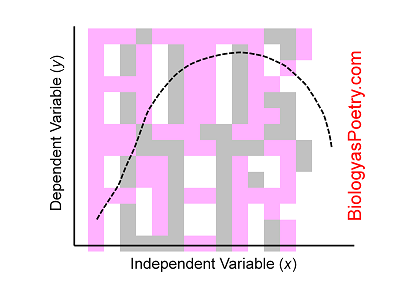# Independent Variable

∞ generated and posted on 2016.08.21 ∞

The conditions that are intentionally varied during an experiment.

You can think of independent variables as "causes" where an experiment represents a cause and effect (and where the dependent variable is the "effect").Figure legend: Shown is a standard graph with independent variable equivalent to the x axis. That is, y, the dependent variable, varies as a function of x, here producing the dotted-line curve.

If you have ever pondered, hey, what would happen if I did "this"? Well, "this" would be the independent variable. And "what would happen" would be the dependent variable.